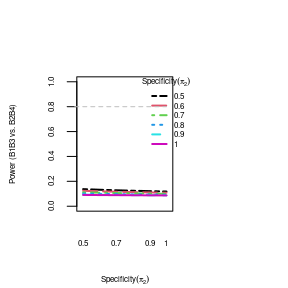# SMART: Dynamic Treatment (DTR)

The purpose of this developing this R package is to quantify and visualize the misclassification effect on mean/variance of dynamic treatment regimens (DTRs) and power comparasion of two DTRs in the setting of sequential multiple assigned randomized trial (SMART).

This vignette describes the use cases of DTRs for the smart package. Most of the trial settings and calculations are handled by the smartDTR() function, where the arguments and settings follow the terminology described in smartSST() function, and the formulae listed in the manuscript He et al (in preparation).

Based on typical two-stage SMART design, the first stage includes two initial treatments denoted as treatment A1 and A2. After making the responder/non-responder designation based on an intermediate outcome, participants are then re-randomized into one of treatments B1 to B8 in treatment stage II. There are then eight possible DTRs: A1B1B3, A1B1B4, A1B2B3, A1B2B4, A2B5B7, A2B5B8, A2B6B7, and A2B6B8. Therefore each DTR includes outcomes from both responder and non-reponder treatments.

Let mu_BsBt=E(Y│B=s or B=t) represents the expected mean response of the DTRs with corresponding variance sigsq_BsBt.

# DTR Calculations

We run smartDTR for mean/variance/relative bias of DTRs (eg. B1B3, B2B4, B5B7). The calculations needed for one run of smartDTR are for two B-levels and the G1,G0 pairing for each B-level. For example, if we want to calculate the mean of B1B3, we will define Barm=c(1,3), then the first number in the setting of G1 represents B1G1, the second number in the setting of G1 represents B3G1, the first number in the setting of G0 represents the B1G0, and the second number in the setting of G0 represents B3G0.

This example supposed that true responders who were correctly assigned into treatment B1 (B1G1) had a better response than true non-responders who were misclassified to B1 (B1G0), and the true non-responders who were correctly classified to treatment B3 (B3G0) had a better response than true responders who were misclassifed to B3 (B3G1).

‘pran_Barm’ represents the probability that participants are assigned into corresponding Blevel. For example, if pran_Blevel=c(0.5, 0.6) under Barm=c(1,3), then the probability of being assigned to B1 group is 0.5, and the probability of being assigned to B3 is 0.6.

mumat13 <- cbind(G1 = c(30, 25), G0 = c(20, 22))
mumat13
     G1 G0
[1,] 30 20
[2,] 25 22
varmat13 <- cbind(G1 = c(16, 16), G0 = c(16, 16))
varmat13
     G1 G0
[1,] 16 16
[2,] 16 16
dtr13 <- smartDTR(mu_Barm = mumat13, sigsq_Barm = varmat13, Barm = c(1, 3), nsubject = 252,
pG_A1 = 0.8, pran_Barm = c(0.5, 0.5))
dtr13$dtrdat # last row shoul dhave sigsq just larger than to input  sens spec n_Barm mu_Barm sigsq_Barm 1 0.5 0.5 63 26.20 27.9600 2 0.5 0.6 63 26.24 27.5424 3 0.5 0.7 63 26.28 27.1216 4 0.5 0.8 63 26.32 26.6976 5 0.5 0.9 63 26.36 26.2704 6 0.5 1.0 63 26.40 25.8400 7 0.6 0.5 63 26.60 28.8400 8 0.6 0.6 63 26.64 28.3904 9 0.6 0.7 63 26.68 27.9376 10 0.6 0.8 63 26.72 27.4816 11 0.6 0.9 63 26.76 27.0224 12 0.6 1.0 63 26.80 26.5600 13 0.7 0.5 63 27.00 29.4000 14 0.7 0.6 63 27.04 28.9184 15 0.7 0.7 63 27.08 28.4336 16 0.7 0.8 63 27.12 27.9456 17 0.7 0.9 63 27.16 27.4544 18 0.7 1.0 63 27.20 26.9600 19 0.8 0.5 63 27.40 29.6400 20 0.8 0.6 63 27.44 29.1264 21 0.8 0.7 63 27.48 28.6096 22 0.8 0.8 63 27.52 28.0896 23 0.8 0.9 63 27.56 27.5664 24 0.8 1.0 63 27.60 27.0400 25 0.9 0.5 63 27.80 29.5600 26 0.9 0.6 63 27.84 29.0144 27 0.9 0.7 63 27.88 28.4656 28 0.9 0.8 63 27.92 27.9136 29 0.9 0.9 63 27.96 27.3584 30 0.9 1.0 63 28.00 26.8000 31 1.0 0.5 63 28.20 29.1600 32 1.0 0.6 63 28.24 28.5824 33 1.0 0.7 63 28.28 28.0016 34 1.0 0.8 63 28.32 27.4176 35 1.0 0.9 63 28.36 26.8304 36 1.0 1.0 63 28.40 26.2400 dtr13$true_mumix
  G1
28.4 
dtr13\$true_sigmix
   G1
26.24 

This is an example of plotting the mean of B1B3 from the above example.

plot.smartDTR(dtr13, metric = "mean", xtype = "spec", mar = c(4, 4, 4, 6), legend.inset = c(-0.2,
0))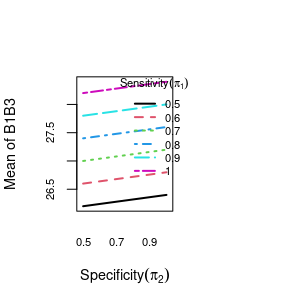plot(dtr13, metric = "mean", relativeBias = TRUE, xtype = "sens", mar = c(4, 4, 4,
6), legend.inset = c(-0.2, 0), ylim = c(0, 10), xlab = "Sens")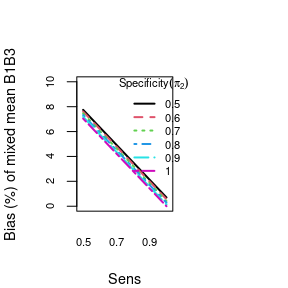This is an example of plotting the variance of B1B3 from the above example.

plot.smartDTR(dtr13, metric = "variance", xtype = "spec", mar = c(4, 4, 4, 6), legend.inset = c(-0.2,
0))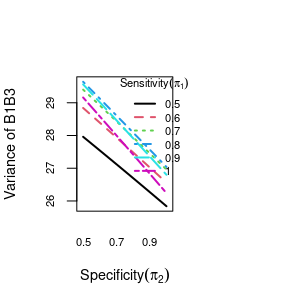plot.smartDTR(dtr13, metric = "variance", xtype = "sens", relativeBias = TRUE)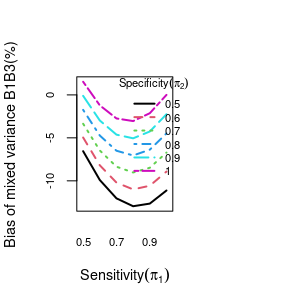## Relative Bias plots

This is an example of plotting the relative mean and relative variance.

plot.smartDTR(dtr13, metric = "mean", relativeBias = TRUE, xtype = "sens", mar = c(4,
4, 4, 6), legend.inset = c(-0.2, 0))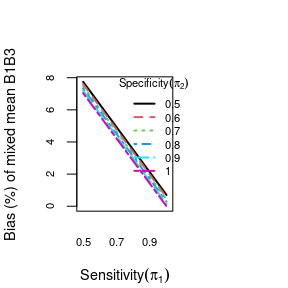plot.smartDTR(dtr13, metric = "variance", relativeBias = TRUE, xtype = "sens", mar = c(4,
4, 4, 6), legend.inset = c(-0.2, 0))## DTR Power comparison-with same initial treatment regimen

To evaluate the power comparison between two DTRs (eg.B1B3 versus B2B4, B1B3 verus B5B7), the calculated results of mean and variance from two DTRs are required. We will use two examples to show the misclassification effects on power based on targeted power of 0.8 (hline=0.8).

The first example shows the power comparison with the same initial treatment regimen A1 (B1B3 versus B2B4). The printed results will include the z-statistics and power under different settings of sensitivity and specificity.

mumat13 <- cbind(G1 = c(30, 35), G0 = c(20, 28))
varmat13 <- cbind(G1 = c(100, 100), G0 = c(100, 100))
# mumat13 <- cbind(G1=c(30,25), G0=c(20,22)) varmat13 <-
# cbind(G1=c(16,16),G0=c(16,16))

dtr13 <- smartDTR(mu_Barm = mumat13, sigsq_Barm = varmat13, Barm = c(1, 3), nsubject = 252)
plot(dtr13, metric = "mean", xtype = "spec", relativeBias = TRUE)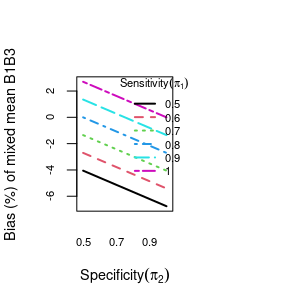plot(dtr13, metric = "variance", xtype = "sens", relativeBias = FALSE)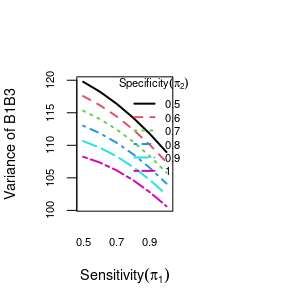mumat24 <- cbind(G1 = c(25, 32), G0 = c(18, 23))
varmat24 <- cbind(G1 = c(100, 100), G0 = c(100, 100))
dtr24 <- smartDTR(mu_Barm = mumat24, sigsq_Barm = varmat24, Barm = c(2, 4), nsubject = 252)
plot(dtr24)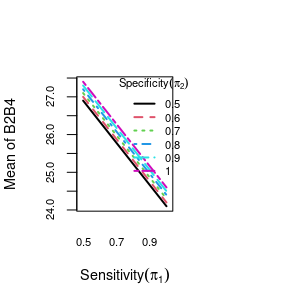pdtr13vs24 <- powerDTR(dtr13, dtr24)
names(pdtr13vs24)
 "powerdat" "Barms"    "alpha"   
# source('../R/powerDTR.R')
plot(pdtr13vs24, mar = c(4, 4, 4, 6), legend.inset = c(-0.2, 0), hline = 0.8)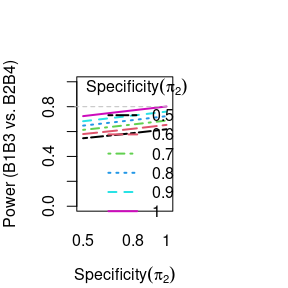plot(pdtr13vs24, alpha = 0.05, xtype = "sens", cex.lab = 0.5, cex.axis = 0.5)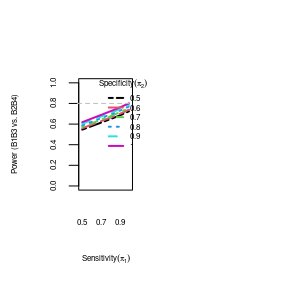## DTR Power comparison-with different initial treatment regimens

The second example shows the power comparison with the different initial treatment regimens (A1B1B3 versus A2B5B7).

mumat13 <- cbind(G1 = c(30, 35), G0 = c(20, 10))
varmat13 <- cbind(G1 = c(100, 100), G0 = c(100, 100))
dtr13 <- smartDTR(mu_Barm = mumat13, sigsq_Barm = varmat13, Barm = c(1, 3), nsubject = 252)

mumat57 <- cbind(G1 = c(29, 32), G0 = c(18, 9))
varmat57 <- cbind(G1 = c(100, 100), G0 = c(100, 100))
dtr57 <- smartDTR(mu_Barm = mumat57, sigsq_Barm = varmat57, Barm = c(5, 7), nsubject = 252)

pdtr13vs57 <- powerDTR(dtr13, dtr57)
names(pdtr13vs57)
 "powerdat" "Barms"    "alpha"   
## Plot DTR Power

plot(pdtr13vs57, mar = c(4, 4, 4, 6), cex.axis = 0.5, cex.lab = 0.5, legend.inset = c(-0.2,
0), hline = 0.8)